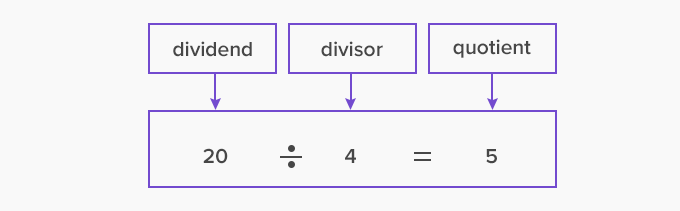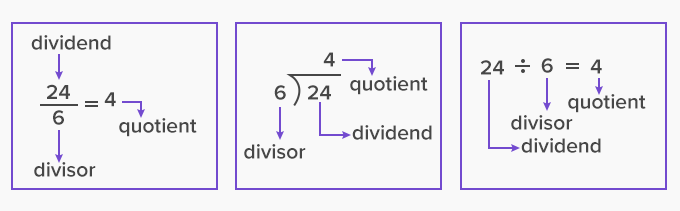# Divisor - Definition with Examples

The Complete K-5 Math Learning Program Built for Your Child

• 30 Million Kids

Loved by kids and parent worldwide

• 50,000 Schools

Trusted by teachers across schools

• Comprehensive Curriculum

Aligned to Common Core

## What is Divisor?

A divisor is a number that divides another number either completely or with a remainder.

A divisor is represented in a division equation as:

 Dividend ÷ Divisor = Quotient.

On dividing 20 by 4 , we get 5. Here 4 is the number that divides 20 completely into 5 parts and is known as the divisor. Its division equation isSimilarly, if we divide 20 by 5, we get 4. Thus, both 4 and 5 are divisors of 20.

## Divisor and factors

The divisor is any number that divides another number. A factor, however, is a divisor that divides the number entirely and leaves no remainder.

So, all factors of a number are its divisors. But not all divisors will be factors.

In the example above, 4 and 5 are the factors of 20.

Whereas, if we divide 20 by 3, it will not completely divide 20. Thus, 3 is not a factor of 20.

Let's consider another example,

15 divided by 5 gives 3. Here, 5 is a divisor.  Also, on dividing 15 divided by 3, we get 5. Here, 3 is a divisor.

The factors and divisors of 15 are:  1, 3, 5, 15

## Finding factors of a number

To find the factors of a number, say 12, we follow the given steps:

1. Start with 1, and write multiplication facts such that the product is 12 such as 1 × 12.

2. Continue the same way to find other multiplication facts till we reach a pair of nearest factors or divisors as given:

1 ×12 , 2×6, 3×4

3. Write down all the numbers in the multiplication fact as these are the divisors of 12.

4. So, the divisors or factors of the number 12 are 1,2,3,4,6 and 12.

## Different ways of representation of divisor

There are different styles of expressing a division equation. In the images below, the various methods of writing a divisor are shown below:Special cases

1. The number 1 is the divisor of all the numbers.

Reason: When the divisor is 1, then the quotient is the same as the dividend.

Look at the given examples,

34  1 = 34

15  1 = 15

2. The number itself is always one of the divisors of the number.

Reason: When the divisor is the same as the dividend, then the answer to such a division is always 1.

Look at the given examples,

34  34 = 1

15  15 = 1

3. When the divisor is a smaller number than the dividend, then the answer is not a whole number but a decimal number.

For example,

510 = 0.5

 Fun Facts If we double the divisor, the quotient will be half.

Won Numerous Awards & Honors